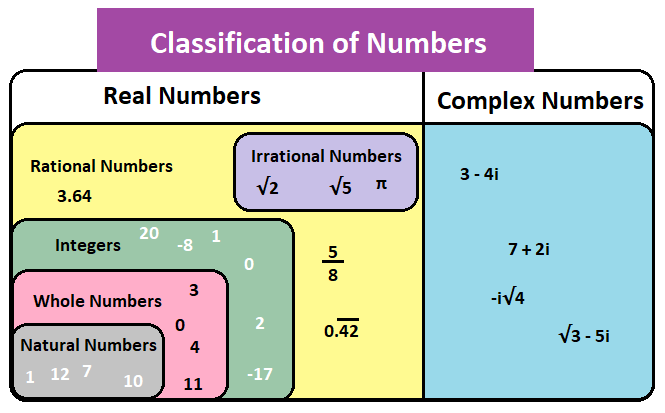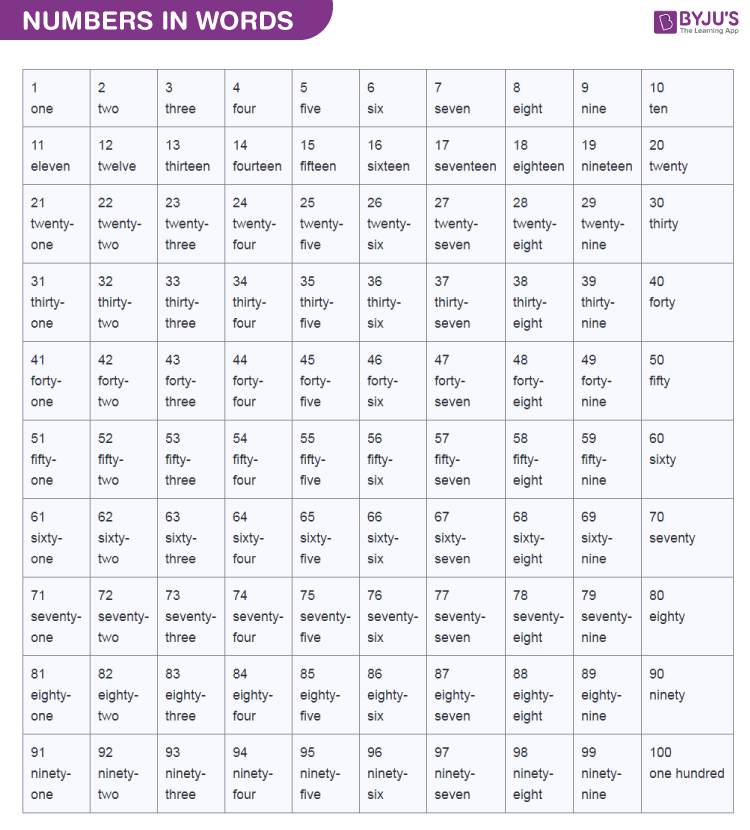We use numbers in our day to day life. They are often called numerals. Without numbers, we cannot do counting of things, date, time, money, etc. Sometimes these numbers are used for measurement and sometimes they are used for labelling. The properties of numbers make them capable of performing arithmetic operations on them.  These numbers are expressed in numeric forms and also in words. For example, 2 is written as two in words, 25 is written as twenty-five in words, etc. Students can practice writing the numbers from 1 to 100 in words to learn more.

There are different types of numbers in Maths, which we learn. They are natural and whole numbers, odd and even numbers, rational and irrational numbers, etc. We will discuss all the types here in this article. Apart from these, the numbers are used in various applications such as forming number series, maths tables, etc.

## Numbers Definition

A number is an arithmetic value used for representing the quantity and used in making calculations. A written symbol like “3” which represents a number is known as numerals. A number system is a writing system for denoting numbers using digits or symbols in a logical manner. The numeral system:

• Represents a useful set of numbers
• Reflects the arithmetic and algebraic structure of a number
• Provides standard representation

We use the digits from 0 to 9 to form all other numbers.

 0 1 2 3 4 5 6 7 8 9

With the help of these digits, we can create infinite numbers.

For example, 12, 3456, 1298, etc

Counting Numbers:

We use numbers to count different things or objects such as 1, 2, 3, 4, etc. Humans have been using numbers to count things from the past thousands of years. For example, there are 7 cows in the field. The counting numbers start from 1 and it goes till infinity.

The Number Zero:

The concept of the number “Zero (0)” place an important role in Mathematics and it is used as a placeholder in the place value number system. The number 0, acts as an additive identity for the real numbers, and other algebraic structures. We use the number “0” to show nothing. For example, there were 3 apples, but now there are none. To represent nothing, we can use zero.

#### Watch The Below Video To Know About Numbers## Types of Numbers

The numbers can be classified  into sets known as the number system. The different types of numbers in maths are:

• Natural Numbers: Natural numbers are known as counting numbers that contain the positive integers from 1 to infinity. The set of natural numbers is denoted as “N” and it includes N = {1, 2, 3, 4, 5, ……….}
• Whole Numbers: Whole numbers are known as non-negative integers and it does not include any fractional or decimal part. It is denoted as “W” and the set of whole numbers includes W = {0,1, 2, 3, 4, 5, ……….}
• Integers: Integers are the set of all whole numbers but it includes a negative set of natural numbers also. “Z” represents integers and the set of integers are Z = { -3, -2, -1, 0, 1, 2, 3}
• Real Numbers: All the positive and negative integers, fractional and decimal numbers without imaginary numbers are called real numbers. It is represented by the symbol “R”.
• Rational Numbers: Any number that can be written as a ratio of one number over another number is written as rational numbers. This means that any number that can be written in the form of p/q. The symbol “Q” represents the rational number.
• Irrational Numbers: The number that cannot be expressed as the ratio of one over another is known as irrational numbers and it is represented by the symbol ”P”.
• Complex Numbers: The number that can be written in the form of a+bi where “a and b” are the real number and “i” is an imaginary number is known as complex numbers “C”.
• Imaginary Numbers: The imaginary numbers are the complex numbers that can be written in the form of the product of a real number and the imaginary unit “i”

Apart from the above, there exist other numbers namely even and odd numbersprime numbers and composite numbers. These can be defined as given below:

Even Numbers: The numbers which are exactly divisible by 2, are called even numbers. These can be positive or negative integers such as -42, -36, -12, 2, 4, 8 and so on.

Odd Numbers: The numbers which are not exactly divisible by 2, are called odd numbers. These can be both positive and negative integers such as -3, -15, 7, 9, 17, 25 and so on.

Prime Numbers: Prime numbers are the numbers that have two factors only. (i.e.,) 1 and the number itself. In other words, the number which is divided by 1 and the number itself is called prime numbers. For example, 2, 3, 5, 7, 11, etc.

Composite Numbers: A composite number is a number that has more than two factors. For example, 4 is a composite number, as the number 4 is divisible by 1, 2, and 4. Other examples of composite numbers are 6, 8, 9, 10, and so on.

Note: The number “1” is neither prime nor composite.

### Numbers Chart

Below is the chart for the classification of numbers:## Numbers in Words

The list of numbers in words from 1 to 100 is given below:## Number Series

In mathematics, the number series consists of a series of numbers in which the next term is obtained by adding or subtracting the constant term to the previous term. For example, consider the series 1, 3, 5, 7, 9, … In this series, the next term is obtained by adding the constant term “2” to the previous term. There are different types of number series namely,

• Perfect Square series
• Two-stage type series
• The odd man out series
• Perfect cube series
• Geometric series
• Mixed series

## Special Numbers

Cardinal Numbers: Cardinal number defines how many of something are there in a list, such as one, five, ten, etc.

Ordinal Numbers: Ordinal numbers explain the position of something in a list, such as first, second, third, fourth, and so on.

Nominal Numbers: Nominal number is used only as a name. It does not denote an actual value or the position of something.

Pi (π): Pi is a special number, which is approximately equal to 3.14159. Pi (π) is defined as the ratio of the circumference of the circle divided by the diameter of the circle.

(i.e.,) Circumference/ Diameter = π = 3.14159.

Euler’s Number (e): Euler’s number is one of the important numbers in Maths, and it is approximately equal to 2.7182818. It is an irrational number and it is the base of the natural logarithm.

Golden Ratio (φ): A golden ratio is a special number and it is approximately equal to 1.618. It is an irrational number and the digits do not follow any pattern.

## Properties of Numbers

The properties of numbers are basically stated for real numbers. The common properties are:

Commutative Property: If  a and b are two real numbers, then according to commutative property;

a+b = b+a

a.b = b.a

Example: 2+3 = 3+2

and 2 × 3 = 3 × 2

Associative Property: If a, b and c are three real numbers, then according to associative property;

(a+b)+c = a+(b+c)

(a.b).c = a.(b.c)

Example: (1+2)+3 = 1+(2+3)

(1.2).3 = 1.(2.3)

Distributive Property: If a, b and c are three real numbers, then according to distributive property;

a × (b + c) = a×b + a×c

Example: 2 × (3 + 4) = 2×3 + 2×4

2 × 7 = 6 + 8

14 = 14

Closure Property: If a number is added to another number, then the result will be a number only, such as;

a+b = c; where a, b and c are three real numbers.

Example: 1+2 = 3

Identity Property: If we add zero to a number or multiply by 1, the number will remain unchanged.

a+0=a

a.1 =a

Example: 5+0 = 5 and 5 x 1 = 5

Additive Inverse: If a number is added to its own negative number, then the result is zero.

a+(-a) = 0

Example: 3+(-3) = 3-3 = 0

Multiplicative Inverse: If a number apart from 0, is multiplied to its own reciprocal then the result is 1.

a x (1/a) = 1

Example: 23 x (1/23) = 1

Zero Product Property: If a.b = 0, then;

either a = 0 or b = 0.

Example: 7 x 0 = 0 or 0 x 6 = 6

Reflexive Property: This property reflects the number itself.

a = a

Example: 9 = 9

The properties which are explained above can vary based on the different types of numbers. To learn the properties of different types of numbers, please check the link given below:

#### Read:Types of Numbers Watch The Below Video To Know The History Of Numbers### Solved Problems

Example 1:

Prove the associative property of addition and multiplication.

Solution:

We know that the associative property of addition and multiplication are:

(a+b)+c = a+(b+c)

(a.b).c = a.(b.c)

Now, assume that a = 2, b= 4 and c = 5

Now, substitute the values in the property

(2+4)+5 = 2+(4+5)

6+5 = 2+9

11 = 11

L.H.S = R.H.S

Hence, (a+b)+c = a+(b+c) is proved.

Proving associative property of multiplication:

(2.4).5 = 2.(4.5)

(8).5 = 2.(20)

40 = 40

L.H.S = R.H.S

Hence, (a.b).c = a.(b.c) is proved.

Example 2:

Solve the given algebraic expression 4.(3+2) using the distributive property

Solution:

Given expression: We know that the distributive property is a × (b + c) = (a×b) + (a×c)

Now, take a= 4, b= 3 and c= 2

Now, substituting values, we get

4.(3+2) =  (a×b) + (a×c)

= (4×3) + (4×2)

= 12+8

= 20

Hence, 4.(3+2) is 20.

Alternate method:

The expression can be solved using BODMAS Rule also

Apply, BODMAS rule in the given expression:4.(3+2)

According to this rule, we have the simplify the value inside the brackets first, so we get

4.(3+2) = 4. 5

Now, multiply the values

4.(3+2) = 20.

## Frequently Asked Questions on Numbers – FAQs

Q1

### What are the different properties of numbers?

The different properties of numbers are:
Associative property
Commutative property
Distributive property
Closure property
Identity Property
Inverse property
Reflexive property
Zero product property

Q2

### Write down the properties for real numbers

The properties of the real number are:
The real numbers obey associative and commutative property
It obeys distributive property under addition and multiplication
The additive identity of real numbers is 0 and the multiplicative identity is 1.

Q3

### What is the difference between rational and irrational numbers?

The rational number is defined as the ratio of two numbers, and it is expressed in the form p/q, where q is not equal to 0. But the irrational number cannot be expressed as the ratio of two numbers.

Q4

### Write down the associative property of numbers.

The associative property of addition is (a+b)+c = a+(b+c)
The associative property of multiplication is (a.b).c = a.(b.c)

Q5

### What are the additive and multiplicative inverse of numbers?

If “x” is a number, the additive inverse of x is -x. The additive inverse property is given as x+ (-x) = 0
If “y” is a number, the multiplicative inverse of y is 1/y. The multiplicative inverse property is given by y. (1/y) = 1.

To know more about numbers and number systems in mathematics, register with BYJU’S – The Learning App to learn with ease.

Quiz on Numbers